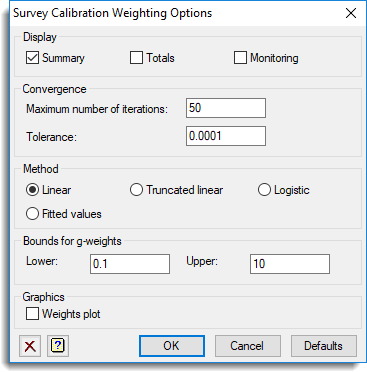1. Home
2. Survey Calibration Weighting Options

# Survey Calibration Weighting Options

Use this to set various options related to calibrating survey weights.## Display

Specifies the output to be displayed.

 Summary Summary of the calibration. Totals Displays estimates of the totals. Monitoring Displays progress of the iterative process.

## Convergence

Controls parameters for the iterative calculations used for the Truncated linear and Logistic methods.

 Maximum number of iterations Maximum number of iterations used in iterative calculations. Tolerance Tolerance for convergence of iterative calculations.

## Method

Specifies the method used for the calibration. Linear calculates estimates equivalent to the usual regression estimates. The g-weights are not restricted and may be negative. Truncated linear restricts the g-weights within the range specified by the Bounds for g-weights settings by replacing extreme values by these bounds. Logistic uses a logistic transformation to ensure that the weights remain within the specified bounds. Fitted values can be produced without any calibration by using the Fitted values setting. This setting avoids having to repeat the full calibration process when analysing additional Y variates.

## Bounds for g-weights

Specifies the bounds for the g-weights when using either the Truncated linear or Logistic methods. The lower bound can be specified using the Lower field and similarly the upper bound can be specified using the Upper field. The default settings are 0.1 for the lower bound and 10 for upper bound, thus allowing the adjusted weights to differ from the initial weights be a factor of 10 in each direction.

## Graphics

Specifies what type of plots can be produced with the analysis. Select Weights plot to produce a plot of the weights.